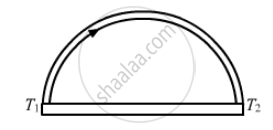Share

# A Semicircular Rod is Joined at Its End to a Straight Rod of the Same Material and the Same Cross-sectional Area. the Straight Rod Forms a Diameter of the Other Rod. the Junctions Are Maintained at V - Physics

ConceptHeat Transfer Conduction

#### Question

A semicircular rod is joined at its end to a straight rod of the same material and the same cross-sectional area. The straight rod forms a diameter of the other rod. The junctions are maintained at different temperatures. Find the ratio of the heat transferred through a cross section of the semicircular rod to the heat transferred through a cross section of the straight rod in a given time.

#### SolutionLet A be the area of cross section and K be the thermal conductivity of the material of the rod.
Let q1 be the rate of flow of heat through a semicircular rod.

Rate of flow of heat is given by

q1 = (dQ) / dt = (K.A (T_1 - T_2 ))/(pi r)
Let qbe the rate of flow of heat through a straight rod.

q_2 = (dQ)/(dt) = (KA (T_1 - T_2))/ (2r)

Ratio of the rate of flow of heat through the 2 rods

= (q1)/(q2) =(2r)/(pir) = 2/pi

Is there an error in this question or solution?

#### APPEARS IN

Solution A Semicircular Rod is Joined at Its End to a Straight Rod of the Same Material and the Same Cross-sectional Area. the Straight Rod Forms a Diameter of the Other Rod. the Junctions Are Maintained at V Concept: Heat Transfer - Conduction.
S